# ISRO Scientist or Engineer Electronics Dec 2017

Instructions

For the following questions answer them individually

Question 61

# The electric field of a linearly polarized electromagnetic wave is given by $$E_{i}=\hat{a_{x}}E_{o}(x,y)e^{-jkz}$$ is incident upon a linearly polarized antenna whose electric field polarization is expressed as $$E_{a}=(\hat{a_{x}}+\hat{a_{y}}) E(r,\theta,\phi)$$.Find the polarization loss factor.Question 62

# A lossless T-junction two way power divider has a source impedance, input transmission line impendence and $$\frac{o}{p}$$ port load impendence of 50 Ω. Find the output characterization impedances so that the input power is divided in a 2:1 ratio.Question 63

# Following circuit implements a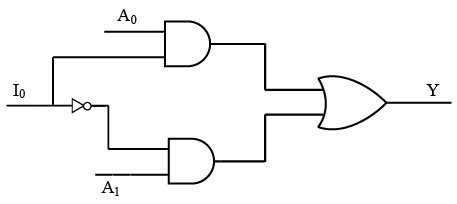Question 64

# The frequency of the output Y is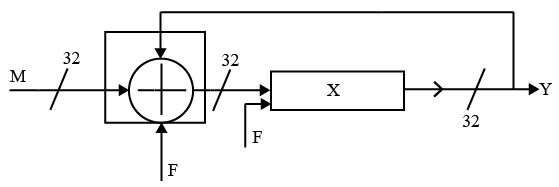F : clock freq.M : inputX : 32 bit registerQuestion 65

# Consider the output A and B with $$I_{0},I_{1},I_{2}$$ and $$I_{3}$$ as input$$A=\overline{I_{3}} \overline{I_{2}} I_{1}+I_{3}$$$$B=\overline{I_{3}} I_{1}+I_{3}$$The above circuit isQuestion 66

#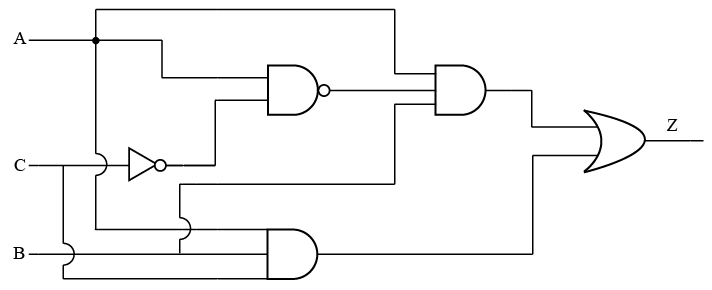The output Z =Question 67

# Consider the shift register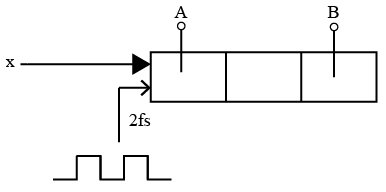The frequency of signal x is fs and the shift register is clocked at the positive edge of 2fs. The time offset between A and B isQuestion 68

# The circuit is formed as shown below. The output S and C implement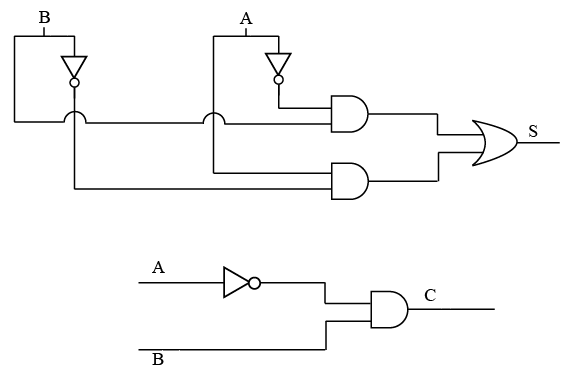Question 69

# What is the value of the register formed from D flip-flops using $$Q_{0},Q_{1}$$, and $$Q_{2}$$ as output $$(Q_{0},Q_{1},Q_{2})$$ after 14 cycles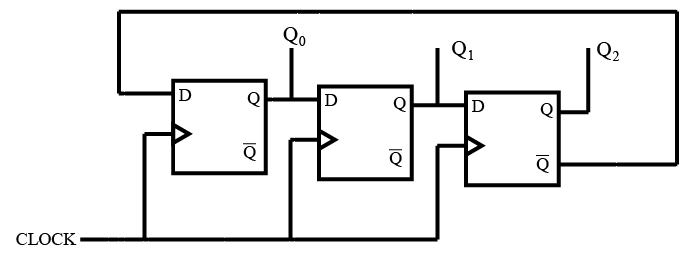Question 70

# The 4:1 Multiplexer implemented as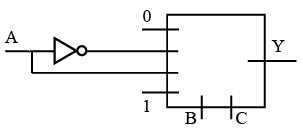OR